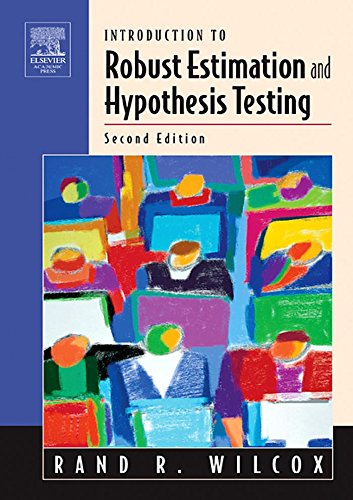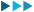Introduction to Robust Estimation and Hypothesis Testing (Statistical Modeling and Decision Science) Textbooks

MRP: 14916.7
1896.287%

Thank you for subscribing. You are just one more step away from getting price alerts. Please go to your email inbox & verify your email id.
View more deals fromx

Price Graph

1. Current price of Introduction to Robust Estimation and Hypothesis Testing (Statistical Modeling and Decision Science) Textbooks is Rs. 1896.2
2. Price of Introduction to Robust Estimation and Hypothesis Testing (Statistical Modeling and Decision Science) Textbooks has dropped to its minimum 5 days ago !
3. The lowest price for Introduction to Robust Estimation and Hypothesis Testing (Statistical Modeling and Decision Science) Textbooks was noted on 2019-06-19 18:07:15
4. Introduction to Robust Estimation and Hypothesis Testing (Statistical Modeling and Decision Science) Textbooks is available at it's all time low price ! You can go for this product now :)
5. Price of Introduction to Robust Estimation and Hypothesis Testing (Statistical Modeling and Decision Science) Textbooks has varied from 1896.2 to 14916.7 in last 1074 days.
6. Percentage Drop shown in the deal is w.r.t MRP of the product. It doesn't depict how good a particular deal is !

Top Deals